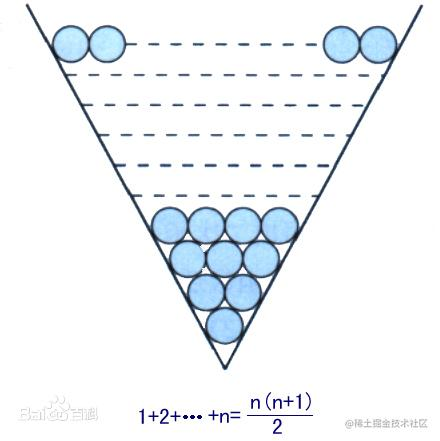# 一、题目描述

Hercy 想要为购买第一辆车存钱。他 每天 都往力扣银行里存钱。

• 每天一道LeetCode题目，开开心心过日子~

# 二、思路分析

• 老规矩，按照做题方法来，先读懂题意，理解示例，找到解题思路，最后再撸成代码~

• 示例一：

• 输入：n = 4
输出：10
解释：第 4 天后，总额为 1 + 2 + 3 + 4 = 10 。
复制代码
• 示例二：

• 输入：n = 10
输出：37
解释：第 10 天后，总额为 (1 + 2 + 3 + 4 + 5 + 6 + 7) + (2 + 3 + 4) = 37 。
注意到第二个星期一，Hercy 存入 2 块钱。
复制代码
• 示例三：

• 输入：n = 20
输出：96
解释：第 20 天后，总额为 (1 + 2 + 3 + 4 + 5 + 6 + 7) + (2 + 3 + 4 + 5 + 6 + 7 + 8) + (3 + 4 + 5 + 6 + 7 + 8) = 96 。
复制代码
• 由上面的示例来看，很明显可以看出来这玩意其实就是多个等差数列计算和而已!

• 等差数列：先搞懂等差数列是个啥？

• 等差数列是常见的一种，如果一个数列从第二项起，每一项与它的前一项的差等于同一个常数，这个数列就叫做等差数列，而这个常数叫做等差数列的公差，公差常用字母d表示。

• 高中数学公式：

• 通项公式推导：
a2-a1=d;a3-a2=d;a4-a3=d……an-a(n-1)=d,将上述式子左右分别相加，得出an-a1=(n-1)*d→an=a1+(n-1)*d。
前n项和公式为：Sn=a1*n+[n*(n-1)*d]/2
Sn=[n*(a1+an)]/2
Sn=d/2*n²+（a1-d/2）*n
注：以上n均属于正整数。
复制代码
•• $\begin{array}{l} a_{n}=a_{1}+(n-1) \times d \\ S_{n}=n a_{1}+\frac{n(n-1)}{2} d, n \in N^{*} \end{array}$
• 看了公式说明，相信读者已经对等差数列有了一定的了解，这里我们再回过头看下存款的题目：

• 当 n<=7时，1+2+3+4+5+6+7
• 当 7<n<=14时，(1+2+3+4+5+6+7)+(2+3+4+5+6+7+8+9)
• 依次类推
• 无疑这个题目我们的目的就是先计算共有几周，也就是偏移量,然后将多个等差数列相加即是结果！

# 三、AC 代码

• 既然读懂了题目，理解了题意，也明白了解法，那么下面我们就用代码来实现它吧！

• public static int totalMoney(int n) {
int week;//星期
int offset;//偏离量，是不是有点玄乎，其实就是计算是第几周，每周比前一周多一元，以此类推
int deposit=0;//存款
for(int i=0;i<n;i++){
week=i/7;//第几周
/**
* 偏移量，这个可以这样理解
* 比如 i=0 offset = 0%7+1=1 证明第一天存了1元
*     i=1 offset = 1%7+1=2 证明第二天存了2元
*     。
*     。
*     。
*     i=7 offset = 7%7+1=2 证明第八天存了2元
*     以此类推~
*/
offset=i%7+1;
deposit+=week+offset;//总存钱数，累加即可，没啥好解释的
}
return deposit;
}
复制代码
• 提交执行结果：• 代码中有详细注释，在这里就不详细解释了~

• 这种题目主要是靠理解，不能一看到立马就去写，一定要写读懂题意，理解示例，理清自己的思路！最后才将自己的想法写成代码来实现它！

# 四、总结

• 好记性不如烂笔头，将自己的想法写出来，下次回顾时会印象更加深刻！
• 其实LeetCode的绝大多数题目都和数学有很深的关系，所以笔者建议，最好回顾下数学的相关知识，不求深入理解，起码要保证记得绝大多数高中的数学知识点即可！
• 最后，笔者的想法只是一种解法，下面推荐几种不错解法，供需要者自行进行研究，笔者在此不再赘述！
• 暴力解法
• 特技解法